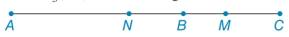Chapter 1.3, Problem 41E### Elementary Geometry for College St...

6th Edition
Daniel C. Alexander + 1 other
ISBN: 9781285195698

#### Solutions

Chapter
Section### Elementary Geometry for College St...

6th Edition
Daniel C. Alexander + 1 other
ISBN: 9781285195698
Textbook Problem
21 views

# Let A B = a and B C = b . Point M is the midpoint of B C ¯ . If A N = 2 3 ( A B ) , find the length of N M ¯ in terms of a and b.To determine

To find:

The length of NM¯ in terms of a and b.

Explanation

Given:

The given figure is,

AB=a and BC=b.

Point M is the midpoint of BC¯ and AN=23(AB).

Approach:

Consider the given figure,

Figure (1)

AB=aAN=23(AB)AN=23a

Also,

### Still sussing out bartleby?

Check out a sample textbook solution.

See a sample solution

#### The Solution to Your Study Problems

Bartleby provides explanations to thousands of textbook problems written by our experts, many with advanced degrees!

Get Started

#### Find more solutions based on key concepts